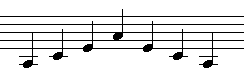Back to start Email meMajor and minor Neil Hawes Home Page
• Try this exercise:
• Sing a major scale: like this
• Now take the top two notes, and "move them" to the bottom; in other words start two notes lower, and end two notes lower
• One way of doing this is to sing down two notes from the start note, then go upwards through the start note like this
• You end up with a foirm of minor scale sounding like this
• Then compare the minor scale with a major scale starting on this lower note: compare major with minor
• Which notes are different? (Hold your mouse over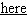to cheat and find out)

• Some music is written using a minor scale rather than a major scale, so below is the some of the theory behind the minor scale

• The definition of a scale, previously hinted at when we first looked at scales, is:
• A scale is a set of notes in order where each "slot" on the stave is occupied by just one note (they are contiguous);
• So far, all scales we have looked at have been based on the major scale;
• However, there are other scales, the most common being the minor scale;
• Different scales differ in the individual gaps between notes, so accidentals are likely to be involved.

• When we looked at accidentals, we saw the notes on a piano keyboard and where the black notes were: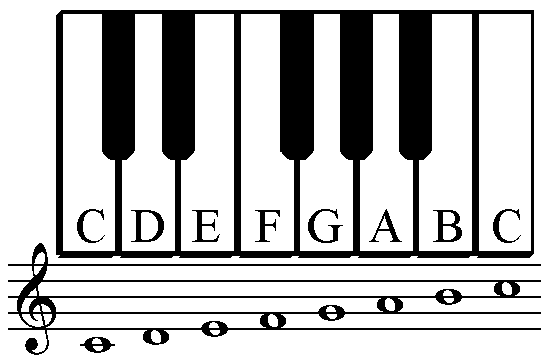• This showed that an octave is made up of 12 equally-spaced intervals, called semitones.

• Another way of specifying a major scale is by listing the intervals between the notes, in a short-hand form where T=tone and S=Semitone:
T T S T T T S
• This says that the gap between C and D is a tone (two semitones), the gap between D and E is a tone, the gap between E and F is a semitone, etc.

• One way of describing the minor scale is that it uses the same notes as the major scale, but starting a third (two notes) below:
• So we can take the intervals of the major scale: T T S T T T S, and move the last two to the front, to make: T S T T S T T.
• The scale starting two notes lower than C looks like this: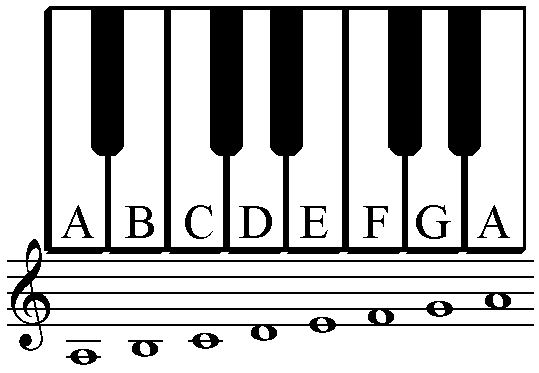• However, there are often variations on the minor scale, depending on the circumstances:
• Often the seventh note, and sometimes the sixth note are sharpened;
• The most common rule is that in an ascending scale both are sharpened, in which case the gaps in the top half of the scale are the same as the major scale (T S T T T T S)
• In a descending scale, both are then naturals;
• Sometimes the sixth can be natural and the seventh sharpened both ascending and descending;
• In this case there is a gap of 3 semitones between the sixth and seventh notes.

• The most noticable difference between the major and minor scales is that the third note is a semitone lower in the minor scale.
• This makes the scale have a mournful or sad feel to it.
• The third note of the scale is in the primary triad, so the triad sounds very different.

• For sight-singing, you need to know the difference in sound between a major and minor scale, and a major and minor triad or arpeggio
• When singing warm-up exercises, the major scale is almost invariably used, but the minor scale can be used just as well
Here's a minor scale to sing: hear it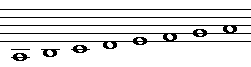Here's a minor scale up and down in rhythm: hear it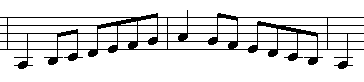And here's a minor arpeggio hear it